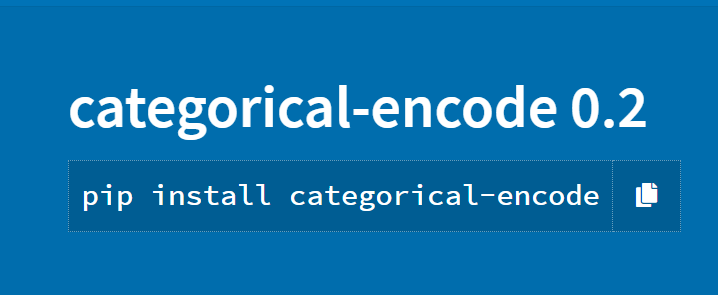# Categorical Data Encoding Python Package (Categorical-Encode)

Original article was published by Nikhil kala on Deep Learning on Medium# Categorical Data Encoding Python Package (Categorical-Encode)

Have you ever pre-processed data? Whenever I am training any Machine Learning Model, this initial step always makes the process monotonous and saps my energy. To remove that hurdle I have published my own Python package for pre-processing data that is categorical in nature. 😬

# Types of Data

We generally deal with two types of Data:

1. Numerical Data:

This type of data is already in numbers and can directly be used in Machine Learning Models.

Eg: Binary Data (0,1)

2. Categorical Data:

In statistics, a categorical variable is a variable that can take on one of a limited, and usually fixed, a number of possible values, assigning each individual or other unit of observation to a particular group or nominal category on the basis of some qualitative property.

Eg: Gender (Male, Female)

# Need for Pre-Processing

The data needs to be pre-processed if the Data which you have is categorical in nature then it needs to be converted into Numerical Data so that it can be used for various statistical and Machine Learning models that work well on numerical data.

Eg:

Data that consists of the States in the United States can be converted to 50 Integer values with each number corresponding to a State.

`{ 1:'Alabama'2:'Alaska’..50:’Wyoming'}`

# Install

To install use the following command in the terminal:

`pip install categorical-encode `

This should install the package in your environment.

# Usage

To use the package just import it using this command:

`from categorical_encode.categorical import categorical`

The function categorical is now ready for use.

Now Let’s take a look at the Parameters we can use.

# Usage Example

Let’s take a Dataset that contains data for Scholarship information. We will apply this function and see all the possible usages which we can get.

This is how the dataset looks like:

This is a categorical data as you can see that all columns except Name can be distributed into classes, It also contains NaN (Empty) values that need to be removed. The Scholarship Received column also needs to be separated because that is the Target Dataframe (The value which needs to be determined after applying Machine Learning Model).

Now on applying this package without any parameters being used:

We can see that this Dataset has been converted to numerical values and can be used for applying various models.

Various Machine Learning models work well on data that is normalized and doesn’t have parameters with many classes(Eg: Names are always different). Now we can use the various parameters to get the full usage of this package: